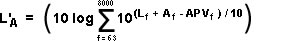USA ▼
Help > FAQ > Protector Octaves

# Hearing Protector Performance - Octave Band Method

The Octave Band method estimates the sound level at the ear, taking into account the frequency content of the noise. This method is more complicated than the NRR, SNR and HML methods, but is the most accurate of the three and better for noise with high tonal or low frequency content.

In order to use the Octave Band method you need the following:

• Octave Band Assumed Protection Values for the Hearing Protector - provided by the manufacturer, usually on the box.
• Sound Level in each Octave Band - measured using a sound level meter with octave band filters

The formula to calculate the level at the ear is:Where:

L'A is the effective A-weighted sound level at the ear
f is the octave band centre frequency in Hz
Lf is the measured octave band sound level in band f
Af is the frequency weighting for octave band f
APVf is the assumed protection value of the hearing protector for octave band f

Some regulations, such as those used in the UK and Europe, recommend adding 4dB to the final result to account for real-world factors, such as the poor fitting of protectors.

## Worked Example

As there are only eight bands of interest for these calculations, it is easy enough to expand this to a usable form:

 Frequency (Hz) f 63 125 250 500 1000 2000 4000 8000 Sound Level (dB) Lf 69.6 74 75.9 77.3 100.1 105.8 77.9 57.1 ⇐ Example data A Weighting Af -26.2 -16.1 -8.6 -3.2 0 1.2 1 -1.1 Protector APV (dB) APVf 8.6 12.2 13.9 15.8 16.4 22.9 24.6 22.7 ⇐ Example data Lf + Af - APVf 34.8 45.7 53.4 58.3 83.7 84.1 54.3 33.3

Now take each of the numbers on the bottom row, divide by 10 and anti-log:

LSUM = 103.48 + 104.57 + 105.34 + 105.83 + 108.37 + 108.41 + 105.43 + 103.33

And finally anti-log the result:

L'A = 10 log (LSUM)

L'A = 86.9 dB

In the EU and the UK the regulations recommend adding a "real-world factor" of 4dB to account for poor fitting of hearing protectors.
In this case L'A = 90.9 dB

So in this example, after all that work, these particular hearing protectors are inadequate.

### Hearing Protection Calculator

NoiseMeters provides a range of online applications for calculating noise exposures along with other noise related calculators. These applications are free to use.

You can use our Octave Band hearing protection calculator to carry out the above calculations and produce a simple report. All you need are the octave band sound level measurements and the octave band APV figures from the hearing protector specifications.

Octave Band Hearing Protection Calculator